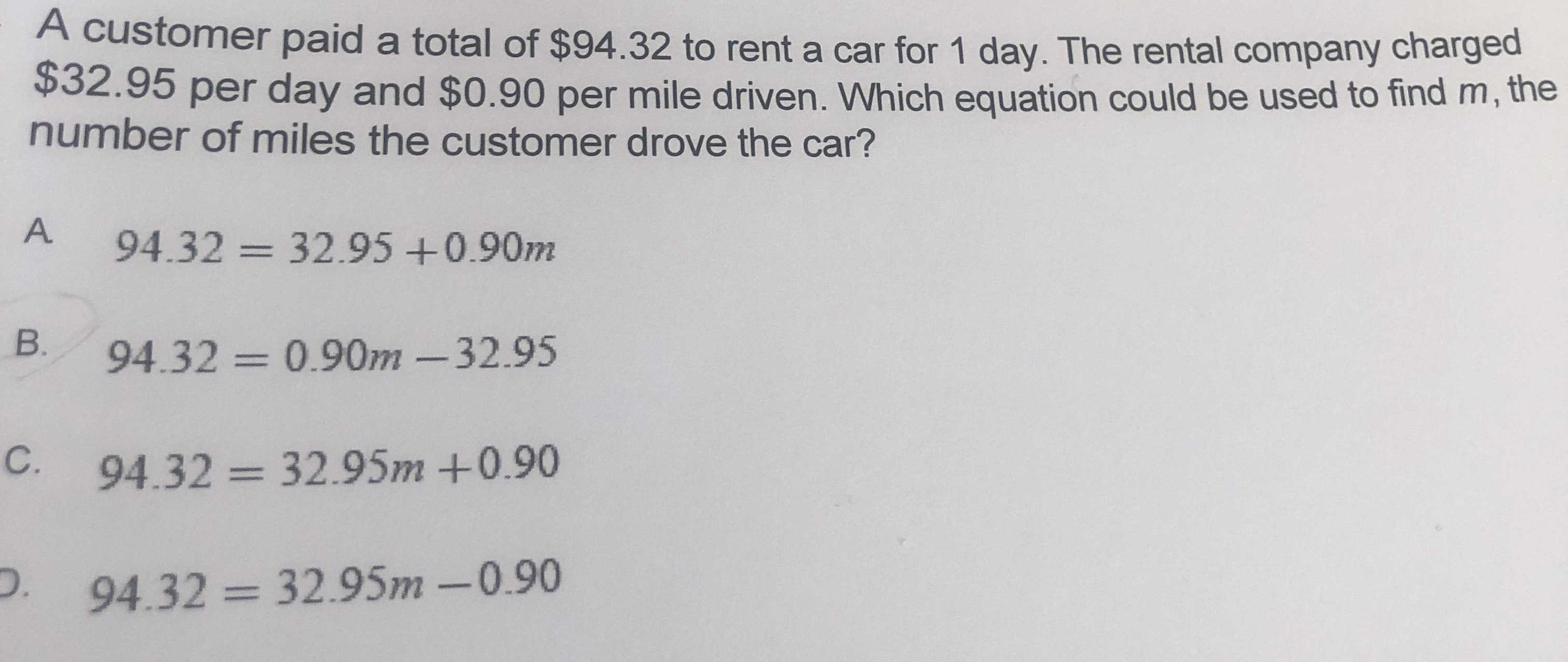### ¿Todavía tienes preguntas de matemáticas?

Pregunte a nuestros tutores expertos
Algebra
PreguntaA customer paid a total of $$\ 94.32$$ to rent a car for $$1$$ day. The rental company charged

$$\ 32.95$$ per day and $$\ 0.90$$ per mile driven. Which equation could be used to find $$m$$ , the number of miles the customer drove the car?

A. $$94.32 = 32.95 + 0.90 m$$

B. $$94.32 = 0.90 m - 32.95$$

C. $$94.32 = 32.95 m + 0.90$$

D. $$94.32 = 32.95 m - 0.90$$